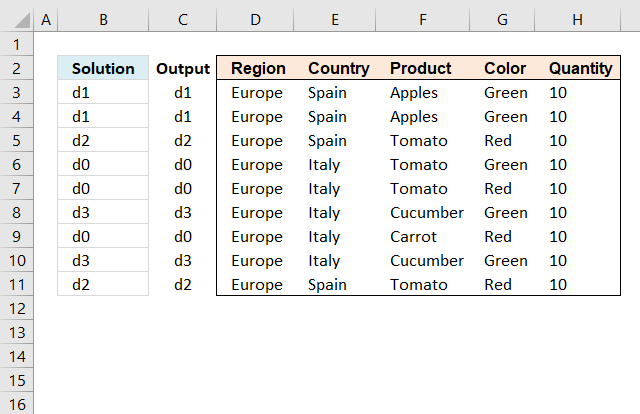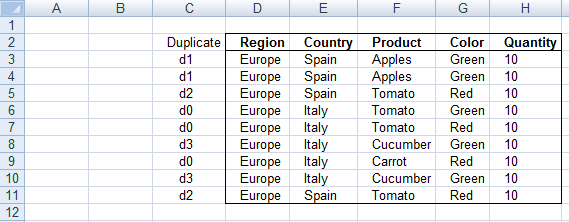Author: Oscar Cronquist Article last updated on March 20, 2019I need to identify the duplicates based on the Columns D:H and put in Column C a small “d” + a running number for the duplicates which are duplicated the same.
If no duplicates only to put in a “d0” for instance.The following array formula assigns unique records the label "d0" and duplicate records the same label

Array formula in cell B3:

=IF(COUNTIFS(\$D\$3:\$D\$11, D3, \$E\$3:\$E\$11, E3, F\$3:\$F\$11, F3, \$G\$3:\$G\$11, G3, \$H\$3:\$H\$11, H3)=1, "d0", IF(COUNTIFS(\$D\$3:D3, D3, \$E\$3:E3, E3, \$F\$3:F3, F3, \$G\$3:G3, G3)=1, "d"&(SUM(1/COUNTIFS(\$D\$3:D3, \$D\$3:D3, \$E\$3:E3, \$E\$3:E3, F\$3:F3, \$F\$3:F3, \$G\$3:G3, \$G\$3:G3, \$H\$3:H3, \$H\$3:H3))-SUM(IF(COUNTIFS(\$D\$3:\$D\$11, \$D\$3:D3, \$E\$3:\$E\$11, \$E\$3:E3, F\$3:\$F\$11, \$F\$3:F3, \$G\$3:\$G\$11, \$G\$3:G3, \$H\$3:\$H\$11, \$H\$3:H3)=1, 1, 0))), INDEX(\$B\$3:B3, MIN(IF(COUNTIFS(D3, \$D\$3:D3, E3, \$E\$3:E3, F3, \$F\$3:F3, G3, \$G\$3:G3, H3, \$H\$3:H3), MATCH(ROW(\$D\$3:D3), ROW(\$D\$3:D3)), "")))))

To enter an array formula, type the formula in a cell then press and hold CTRL + SHIFT simultaneously, now press Enter once. Release all keys.

The formula bar now shows the formula with a beginning and ending curly bracket telling you that you entered the formula successfully. Don't enter the curly brackets yourself.

#### How to copy array formula

1. Select cell B3.
2. Copy cell (Ctrl + c).
3. Select cell range B4:B11.
4. Paste to cell range (Ctrl + v).

### Explaining formula in cell B3#### Step 1 - Identify if record is unique

The COUNTIFS function allows you to count cells based on multiple conditions.

COUNTIFS(\$D\$3:\$D\$11, D3, \$E\$3:\$E\$11, E3, F\$3:\$F\$11, F3, \$G\$3:\$G\$11, G3, \$H\$3:\$H\$11, H3)=1

becomes

COUNTIFS({"Europe"; "Europe"; "Europe"; "Europe"; "Europe"; "Europe"; "Europe"; "Europe"; "Europe"},"Europe",{"Spain"; "Spain"; "Spain"; "Italy"; "Italy"; "Italy"; "Italy"; "Italy"; "Spain"},"Spain",{"Apples"; "Apples"; "Tomato"; "Tomato"; "Tomato"; "Cucumber"; "Carrot"; "Cucumber"; "Tomato"},"Apples",{"Green"; "Green"; "Red"; "Green"; "Red"; "Green"; "Red"; "Green"; "Red"},"Green",{10; 10; 10; 10; 10; 10; 10; 10; 10},10)=1

becomes

2=1

and returns the boolean value FALSE. It means that the record is not unique, in other words, there is  at least one other duplicate record.

#### Step 2 - Return "d0" if record is unique

The IF function has three arguments, the first one must be a logical expression. If the expression evaluates to TRUE then one thing happens (argument 2) and if FALSE another thing happens (argument 3).

The previous step returns TRUE or FALSE an we are going to use that in our IF function.

IF(COUNTIFS(\$D\$3:\$D\$11, D3, \$E\$3:\$E\$11, E3, F\$3:\$F\$11, F3, \$G\$3:\$G\$11, G3, \$H\$3:\$H\$11, H3)=1, "d0", remaining_formula)

becomes

IF(FALSE, "d0", remaining_formula)

and the calculation continues in the last IF argument.

#### Step 3 - Is record the first of all duplicate records?

COUNTIFS(\$D\$3:D3, D3, \$E\$3:E3, E3, \$F\$3:F3, F3, \$G\$3:G3, G3)=1

becomes

1=1

and returns TRUE.

#### Step 4 - Calculate number if record is first record

IF(COUNTIFS(\$D\$3:D3, D3, \$E\$3:E3, E3, \$F\$3:F3, F3, \$G\$3:G3, G3)=1, "d"&(SUM(1/COUNTIFS(\$D\$3:D3, \$D\$3:D3, \$E\$3:E3, \$E\$3:E3, F\$3:F3, \$F\$3:F3, \$G\$3:G3, \$G\$3:G3, \$H\$3:H3, \$H\$3:H3))-SUM(IF(COUNTIFS(\$D\$3:\$D\$11, \$D\$3:D3, \$E\$3:\$E\$11, \$E\$3:E3, F\$3:\$F\$11, \$F\$3:F3, \$G\$3:\$G\$11, \$G\$3:G3, \$H\$3:\$H\$11, \$H\$3:H3)=1, 1, 0))), remaining_formula)

becomes

IF(TRUE, "d"&(SUM(1/COUNTIFS(\$D\$3:D3, \$D\$3:D3, \$E\$3:E3, \$E\$3:E3, F\$3:F3, \$F\$3:F3, \$G\$3:G3, \$G\$3:G3, \$H\$3:H3, \$H\$3:H3))-SUM(IF(COUNTIFS(\$D\$3:\$D\$11, \$D\$3:D3, \$E\$3:\$E\$11, \$E\$3:E3, F\$3:\$F\$11, \$F\$3:F3, \$G\$3:\$G\$11, \$G\$3:G3, \$H\$3:\$H\$11, \$H\$3:H3)=1, 1, 0))), remaining_formula)

becomes

IF(TRUE, "d"&(SUM(1/1)-SUM(IF(COUNTIFS(\$D\$3:\$D\$11, \$D\$3:D3, \$E\$3:\$E\$11, \$E\$3:E3, F\$3:\$F\$11, \$F\$3:F3, \$G\$3:\$G\$11, \$G\$3:G3, \$H\$3:\$H\$11, \$H\$3:H3)=1, 1, 0))), remaining_formula)

becomes

IF(TRUE, "d"&(SUM(1/1)-SUM(IF(2=1, 1, 0))), remaining_formula)

becomes

IF(TRUE, "d"&(SUM(1/1)-SUM(IF(FALSE, 1, 0))), remaining_formula)

becomes

IF(TRUE, "d"&(1-0), remaining_formula)

becomes

IF(TRUE, "d"&1, remaining_formula)

and returns "d1" in cell B3.

#### Step 5 - Get value from the first duplicate record

This part of the formula is rund if the record is NOT the first duplicate of a specific record in the list, it finds the first record and returns that value.

In cell B4 the formula changes to the following line because of absolute and relative cell references.

INDEX(\$B\$3:B4, MIN(IF(COUNTIFS(D4, \$D\$3:D4, E4, \$E\$3:E4, F4, \$F\$3:F4, G4, \$G\$3:G4, H4, \$H\$3:H4), MATCH(ROW(\$D\$3:D4), ROW(\$D\$3:D4)), ""))))

becomes

INDEX(\$B\$3:B4, MIN(IF({1;1}, MATCH(ROW(\$D\$3:D4), ROW(\$D\$3:D4)), ""))))

becomes

INDEX(\$B\$3:B4, MIN(IF({1;1}, {1; 2}, ""))))

becomes

INDEX(\$B\$3:B4, MIN({1; 2}))

becomes

INDEX(\$B\$3:B4, 1)

becomes

INDEX({"d1";"d1"}, 1)

and returns "d1" in cell B4.

The INDEX function returns a value based on a cell reference and column/row numbers.

### Get the Excel fileGroup-duplicates-1.xlsxx